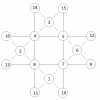BrainDen.com - Brain Teasers

Question

By connecting the eight vertices of an octagon (with horizontal, vertical

and 45-degree lines, ignoring the perimeter) we define eight rows having

either four or five points of intersection.

Place sixteen consecutive (positive) integers on these points such that

1. The sums along all eight rows are the same.
2. The common sum is the smallest possible.

A harder problem is to create the largest possible common sum.Recommended Posts

• 0EDIT: assuming all integers must be positive.

Edited by Rainman

Share on other sites
• 0

Let the 16 numbers be n+1 through n+16, for some natural number n. Take the four rows of 5 numbers each, and add them together. You will get four numbers counted twice, and the rest counted once. The minimum total sum is (n+1)+(n+2)+(n+3)+...+(n+16)+(n+1)+(n+2)+(n+3)+(n+4) = 20n+146. Since all of these rows must have the same sum, the minimum sum for one of them is ceiling((20n+146)/4) = 5n+37. So the lowest possible sum per row is 37, for n=0, which is exactly what I found in my previous post.

Share on other sites
• 0

Here's a solution for the maximum

common sum (41) using the integers
from 1 to 16:
....13..07....
......09......
04..11..14..12
..06......03..
08..16..15..02
......10......
....01..05....

I've found 8 distinct solutions (up
to symmetries) for a common sum of 41.

Proof that 41 is maximal:
Let S be the common sum and let C
be the sum of the four closest
numbers to the center of the octagon.
Then, since the numbers making C
are used in three of the sums, whereas
all the other numbers are in only two
of the sums,
8*S - C = 32*n + 16*17
(n comes from the consecutive integers
being n+1, n+2, n+3, ..., n+16).
In this case, n=0. Now, we rearrange
to solve for S to get
S = 4*n + 34 + (C/8)
which means that C must be divisible
by 8. The largest C possible by
adding four different numbers in the
set {1,2,3,...,16} is 56. This makes
S in our case to be 41.

Share on other sites
• 0

OP doesn't require the numbers 1 through 16, just that the numbers are consecutive.

Again, let the numbers be n+1 through n+16 for some natural number n. If we add together the four rows with four numbers each, we get four numbers counted twice, eight numbers counted once, and four numbers counted nonce. The maximum sum of this is (n+5)+(n+6)+(n+7)+...+(n+16)+(n+13)+(n+14)+(n+15)+(n+16) = 16n+184, which makes the maximum individual sum (16n+184)/4 = 4n+46. Considering that the minimum sum for a five number row is 5n+37, and the fact that the sums must be equal, we get 0 = (five number sum)-(four number sum) >/= (5n+37)-(4n+46) = n-9, which means n </= 9. However, we can't have equality because the numbers to be counted nonce in the four number rows are counted twice in the five number rows. For the five number rows to have a sum of 5n+37, or a total sum of 20n+148, the numbers counted twice must have a sum of 4n+12. But for the four number rows to have a sum of 4n+46, or a total sum of 16n+184, the same numbers must have a sum of 4n+10. This is a contradiction. So n<9. For n=8, I think it's possible to achieve a sum of 77 per row, but I have yet to find an example.

Share on other sites
• 0

Here are two such octagons for n=8. I believe there are no others

up to symmetries.
....18..20....
......10......
21..19..22..15
..11......14..
17..24..23..13
......09......
....16..12....
....20..21....
......09......
17..19..23..18
..12......10..
16..24..22..15
......13......
....14..11....

•1

Join the conversation

You can post now and register later. If you have an account, sign in now to post with your account.×   Pasted as rich text.   Paste as plain text instead

Only 75 emoji are allowed.

×   Your previous content has been restored.   Clear editor

×   You cannot paste images directly. Upload or insert images from URL.

×